Prime Factors Generating the Prime Number Spiral

An interesting prime number spiral was discovered in 1963 by Stanislaw M. Ulam, and is now called "the Ulam spiral". It reveals a strange property of the prime numbers.

A positive integer (1, 2, 3, ... ) greater than 1 is called prime if its only divisors are 1 and itself. The first few prime numbers are 2, 3, 5, 7, 11, 13, 17, 19, 23, 29, 31, 37, 41, 43, ... The series is infinite, since there is no largest prime number. The proof of this goes back to the ancient Greek mathematician Euclid.

The Ulam spiral is constructed as follows: Consider a rectangular grid. We start with the central point and arrange the positive integers in a spiral fashion (anticlockwise) as at below left. The prime numbers are then marked (here with blue boxes). Since the primes occur in an irregular manner in the sequence of numbers one might expect that in this grid they would occur more or less at random. But, on the contrary, there is a clear tendency for the prime numbers to form diagonal lines. This can be seen more clearly in the image below right, in which each of the 70,255 pixels corresponds to a position in the number sequence and the primes are marked by white pixels. This pattern is puzzling, since no explanation has been given for why the prime numbers should line up in this way.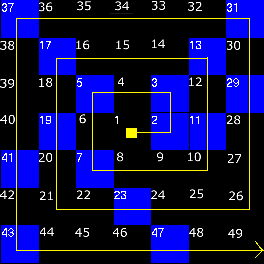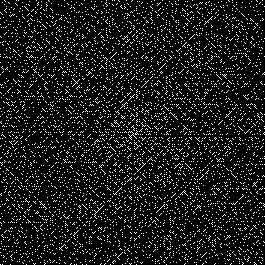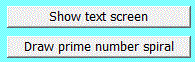After specifying the start and end number, e.g. 1 and 5625, click on the Draw prime number spiral button to obtain: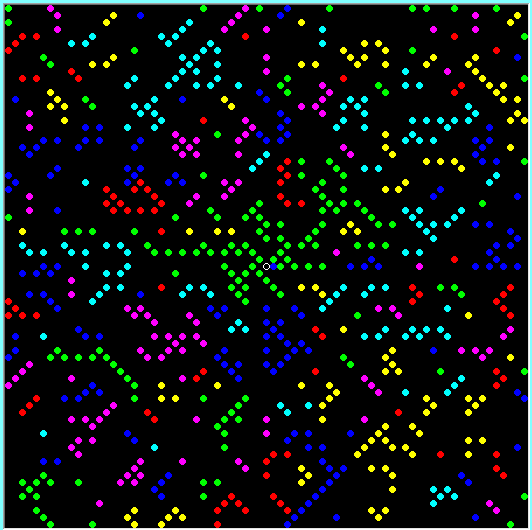The white circle marks the center of the spiral. Clearly the primes tend to line up on the diagonals. This phenomenon persists even when the central number is not 1. And it also persists (to a slightly lesser degree) for very large primes.

The start and end numbers can be (almost) anything (within limits), but the size of the disks depends on the range of numbers, and if the range of numbers is less than the graphic screen will hold then it will not be filled entirely. For example, specifying start and end numbers as 8000 and 16,000 produces the following: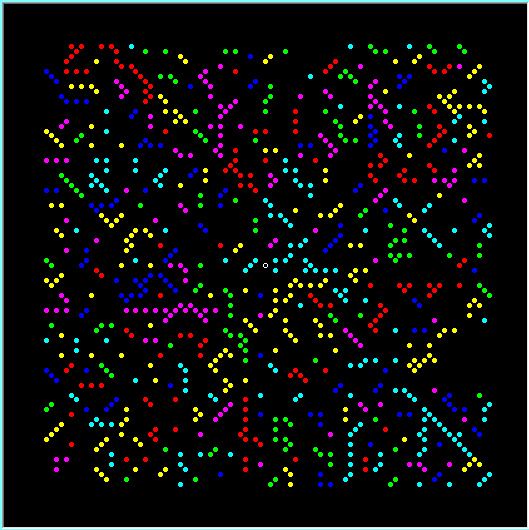Specifying start and end numbers as 8000 and 200,000 produces the following: